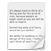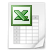A one dimensional Matlab 'model' (time invariant) to calculate carbon export attenuation profiles from measured (surface) particles sinking velocity and (surface) carbon-specific respiration rates. The data and the model equation have been used in Bach et al., 2019

A similar version of the model was already used in one of our previous papers (Bach et al., 2016).Use this Matlab script to calculate attenuation profiles
Bach_model.m
Text Document 6.2 KBDepth and temperature profile
This is needed as an input for the Matlab script.
depth_temp_high_res.csv
Comma Separated Value File 18.6 KBSinking velocity and carbon-specific respiration values
This is needed as an input to understand what was going on in our 2014 Gran Canaria study. However, you can also scroll down in the Matlab script and look for a routine where you can just randomly play around with sinking velocity and respiration values. In this case you do not need this data file.
RLS_data_GC2_model.csv
Comma Separated Value File 2.1 KB